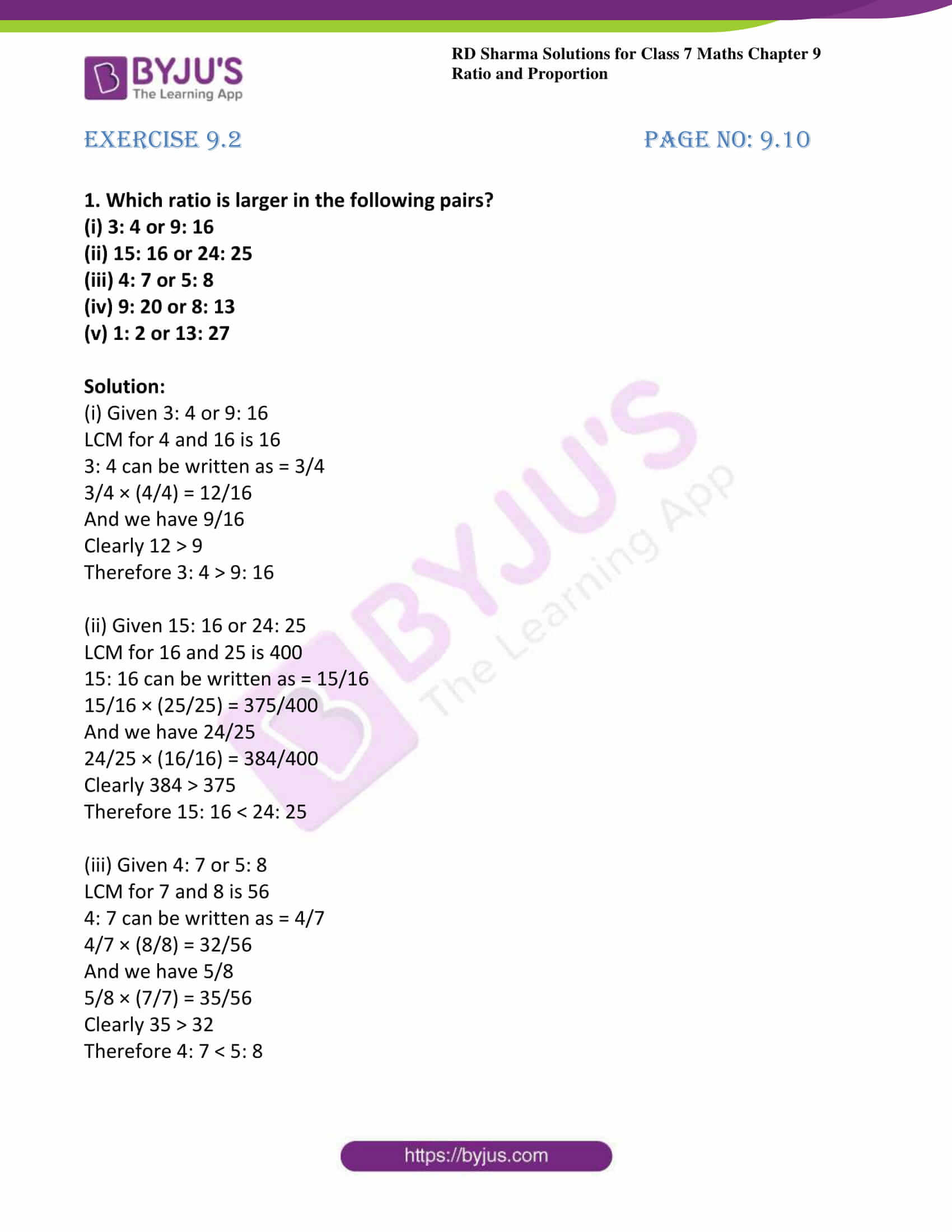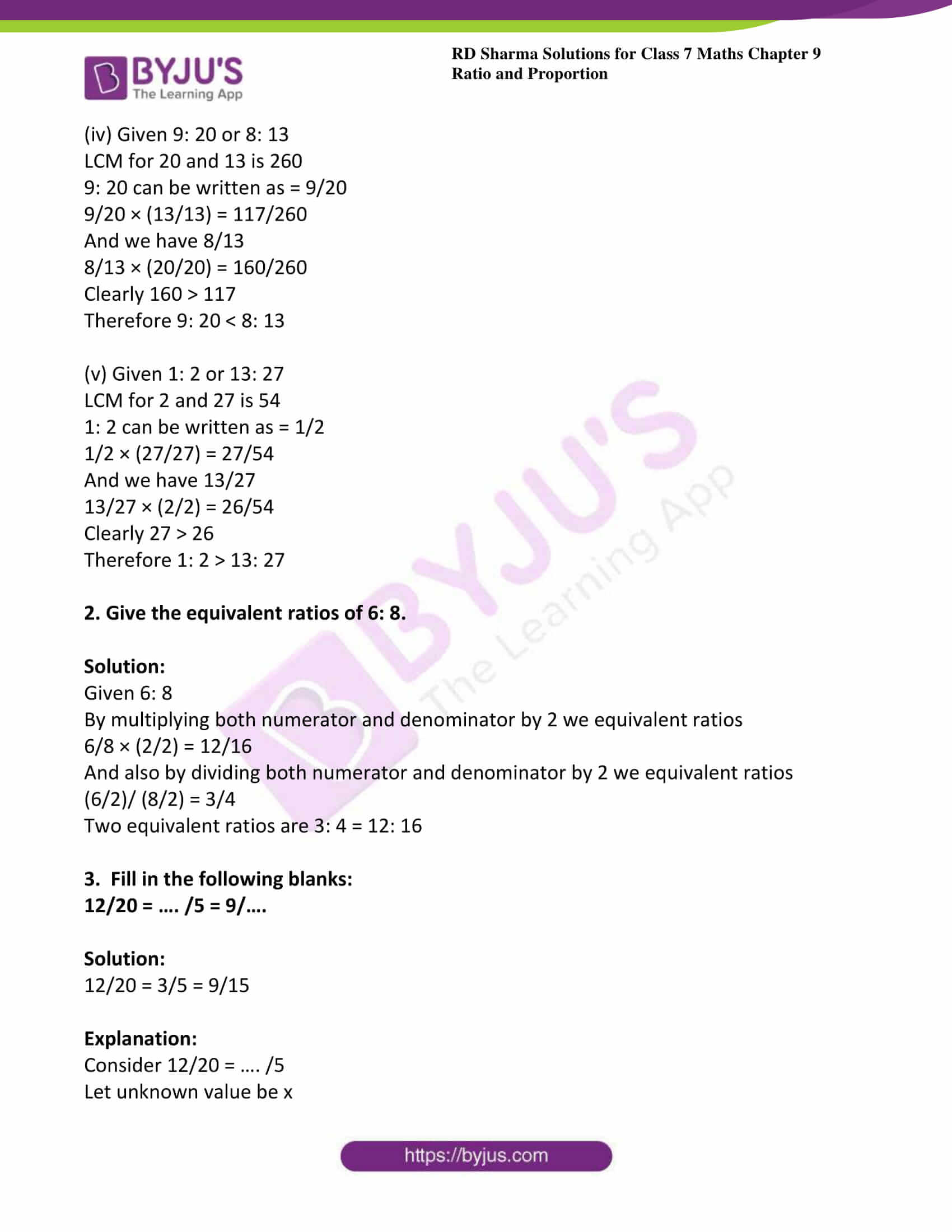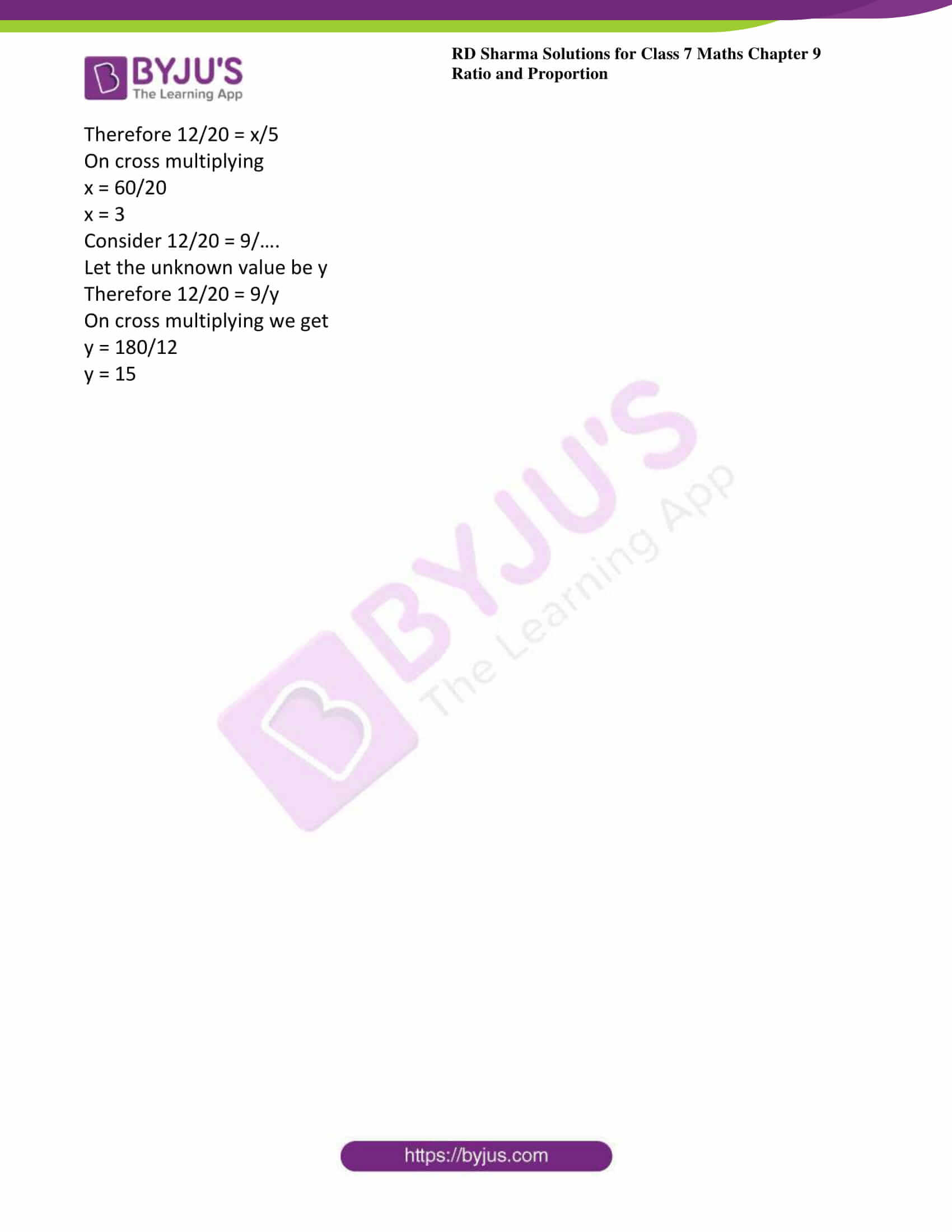# RD Sharma Solutions For Class 7 Maths Exercise 9.2 Chapter 9 Ratio And Proportion

RD Sharma Solution for Class 7 Maths Exercise 9.2 of Chapter 9 Ratio and Proportion are provided in simple PDF format. Students can easily download the PDF from the given links. BYJU’S subject experts formulated answers for all questions present in this exercise briefly. To excel in exams students must practice RD Sharma Solutions for Class 7 Maths. This exercise deals with the comparison of ratios by making denominators the same. It also deals with the equivalent ratio defined as a ratio obtained by multiplying or dividing the numerator and denominator of a given ratio by the same number.

## Download the PDF of RD Sharma Solutions For Class 7 Maths Chapter 9 – Ratio and Proportion Exercise 9.3### Access answers to Maths RD Sharma Solutions For Class 7 Chapter 9 – Ratio and proportion Exercise 9.2

1. Which ratio is larger in the following pairs?

(i) 3: 4 or 9: 16

(ii) 15: 16 or 24: 25

(iii) 4: 7 or 5: 8

(iv) 9: 20 or 8: 13

(v) 1: 2 or 13: 27

Solution:

(i) Given 3: 4 or 9: 16

LCM for 4 and 16 is 16

3: 4 can be written as = 3/4

3/4 × (4/4) = 12/16

And we have 9/16

Clearly 12 > 9

Therefore 3: 4 > 9: 16

(ii) Given 15: 16 or 24: 25

LCM for 16 and 25 is 400

15: 16 can be written as = 15/16

15/16 × (25/25) = 375/400

And we have 24/25

24/25 × (16/16) = 384/400

Clearly 384 > 375

Therefore 15: 16 < 24: 25

(iii) Given 4: 7 or 5: 8

LCM for 7 and 8 is 56

4: 7 can be written as = 4/7

4/7 × (8/8) = 32/56

And we have 5/8

5/8 × (7/7) = 35/56

Clearly 35 > 32

Therefore 4: 7 < 5: 8

(iv) Given 9: 20 or 8: 13

LCM for 20 and 13 is 260

9: 20 can be written as = 9/20

9/20 × (13/13) = 117/260

And we have 8/13

8/13 × (20/20) = 160/260

Clearly 160 > 117

Therefore 9: 20 < 8: 13

(v) Given 1: 2 or 13: 27

LCM for 2 and 27 is 54

1: 2 can be written as = 1/2

1/2 × (27/27) = 27/54

And we have 13/27

13/27 × (2/2) = 26/54

Clearly 27 > 26

Therefore 1: 2 > 13: 27

2. Give the equivalent ratios of 6: 8.

Solution:

Given 6: 8

By multiplying both numerator and denominator by 2 we equivalent ratios

6/8 × (2/2) = 12/16

And also by dividing both numerator and denominator by 2 we equivalent ratios

(6/2)/ (8/2) = 3/4

Two equivalent ratios are 3: 4 = 12: 16

3. Fill in the following blanks:

12/20 = …. /5 = 9/….

Solution:

12/20 = 3/5 = 9/15

Explanation:

Consider 12/20 = …. /5

Let unknown value be x

Therefore 12/20 = x/5

On cross multiplying

x = 60/20

x = 3

Consider 12/20 = 9/….

Let the unknown value be y

Therefore 12/20 = 9/y

On cross multiplying we get

y = 180/12

y = 15